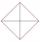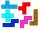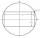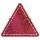# Right triangle - math word problems

1. Triangular prismPlane passing through the edge AB and the center of segmet CC' of regular triangular prism ABCA'B'C', has angle with base 22 degrees, |AB| = 6 cm. Calculate the volume of the prism.
2. Hexagon 5The distance of parallel sides of regular hexagonal is 61 cm. Calculate the length of the radius of the circle described to this hexagon.
3. CosineThe point (8, 6) is on the terminal side of angle θ. cos θ = ?
4. CathetiThe hypotenuse of a right triangle is 41 and the sum of legs is 49. Calculate the length of its legs.In the square ABCD point P is in the middle of the DC side and point Q in the middle pages AD. If the area of quadrilateral BQPC is 49 cm2, what is the area of ABCD?
6. Cone and the ratioRotational cone has a height 23 cm and the ratio of the base surface to lateral surface is 7: 9. Calculate a surface of the base and the lateral surface.
7. SailsWe known heights 220, 165 and 132 of sail. It has triangular shape. What is the surface of the sail?
8. RT 11Calculate the area of right tirangle if its perimeter is p = 45 m and one cathethus is 20 m long.8.3 meters long ladder is leaning against the wall of the well, and its lower end is 1.2 meters from this wall. How high from the bottom of a well is the top edge of the ladder?
10. DistanceWha is the distance between the origin and the point (18; 22)?
11. LegDetermine the area of a trapezoid with bases 71 and 42 and height is 4 shorter than the its leg.
12. CosineCalculate the cosine of the smallest internal angle in a right-angled triangle with cathetus 3 and 8 and with the hypotenuse 8.544.
13. Inscribed rectangleThe circle area is 216. Determine the area of inscribed rectangle with one side 5 long.
14. ISO trapeziumCalculate area of isosceles trapezoid with base 95 long, leg 27 long and with the angle between the base and leg 70 degrees.
15. Chord - TS v2The radius of circle k measures 87 cm. Chord GH = 22 cm. What is TS?
16. 30-60-90The longer leg of a 30°-60°-90° triangle measures 5. What is the length of the shorter leg?
17. Chord - TSThe radius of circle k measures 68 cm. Arc GH = 47 cm. What is TS?
18. Isosceles IVIn an isosceles triangle ABC is |AC| = |BC| = 13 and |AB| = 10. Calculate the radius of the inscribed (r) and described (R) circle.
19. EQL triangleCalculate inradius and circumradius of equilateral triangle with side a=77 cm.
20. FlowerbedFlowerbed has the shape of an isosceles obtuse triangle. Arm has a size 5.5 meters and an angle opposite to the base size is 94°. What is the distance from the base to opposite vertex?

Do you have an interesting mathematical word problem that you can't solve it? Enter it, and we can try to solve it.

We will send a solution to your e-mail address. Solved examples are also published here. Please enter the e-mail correctly and check whether you don't have a full mailbox.

Please do not submit problems from current active competitions such as Mathematical Olympiad, correspondence seminars etc...